# RS Aggarwal Solutions for Class 8 Maths Chapter 8 - Linear Equations Exercise 8A

To score high in the board exams, students can view and download the RS Aggarwal Solutions for the Exercise 8A of Class 8 Maths Chapter 8, Linear Equations from the link available here. BYJU’S experts have solved the questions present in this Exercise, and this will help them more comfortably. By practising the RS Aggarwal Solutions for class 8, students will be able to grasp the concepts flawlessly. It also helps in developing their confidence, which plays an essential role in their examinations. Hence, students whose intention is to score high in the exams are advised to go through RS Aggarwal Solutions for Class 8.

## Download PDF of RS Aggarwal Solutions for Class 8 Maths Chapter 8 – Linear Equations Exercise 8A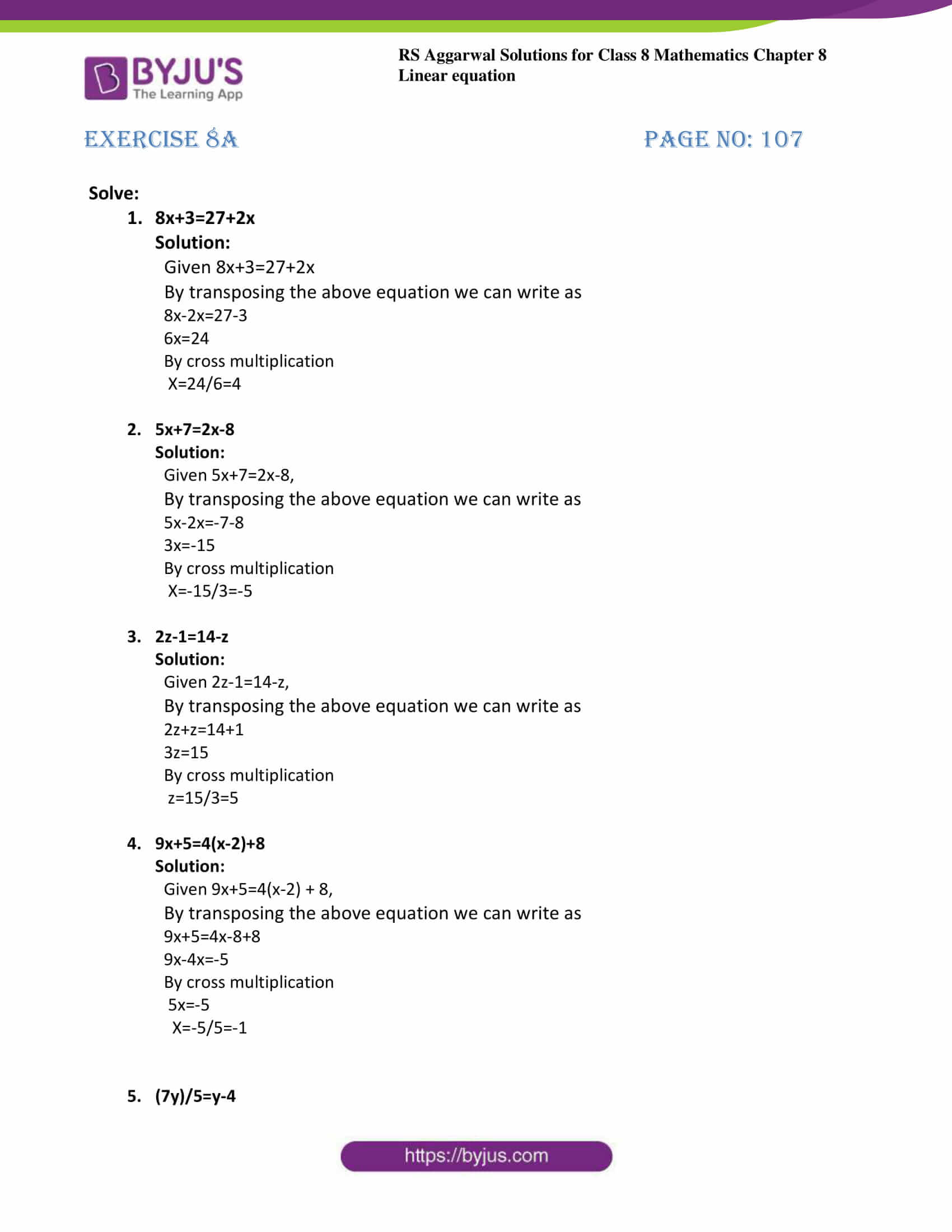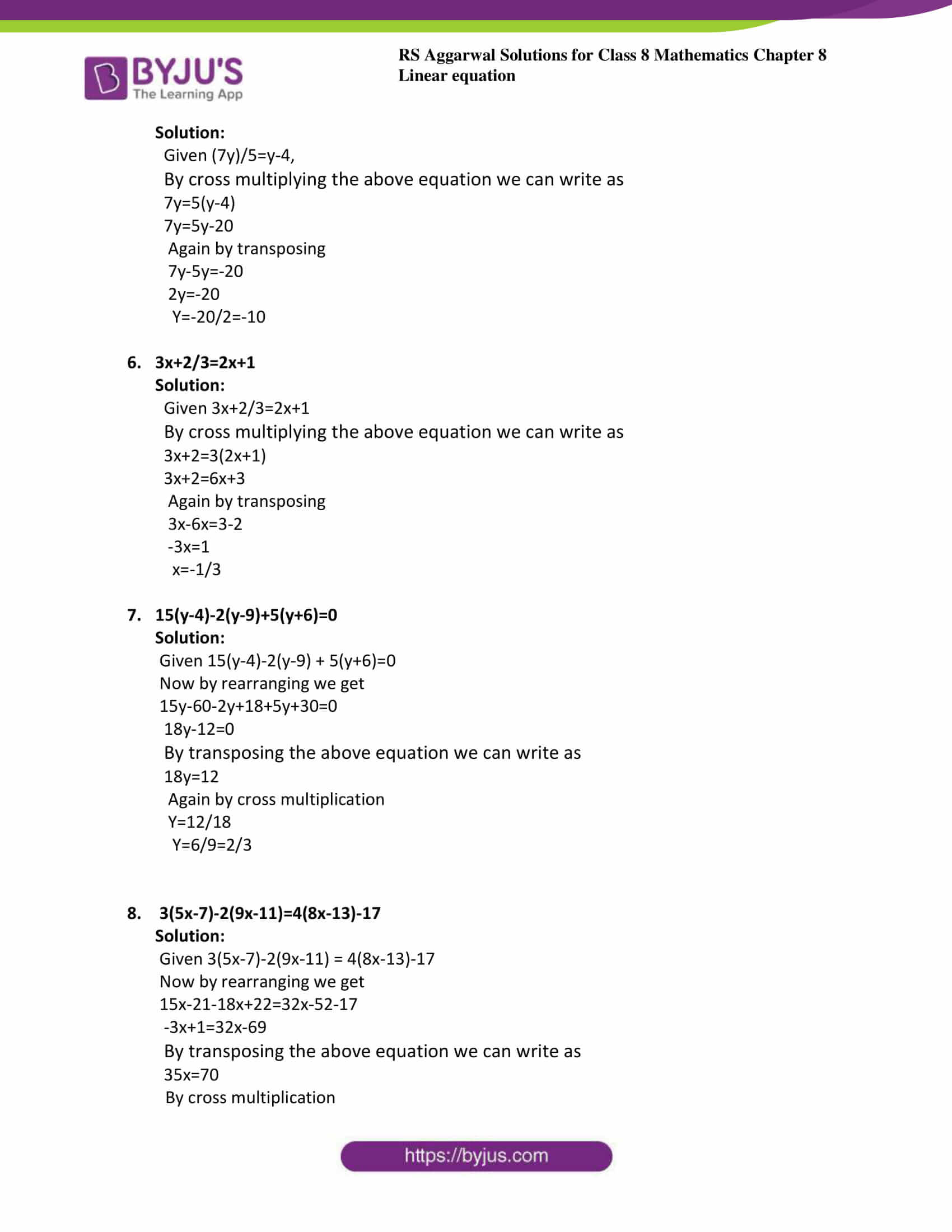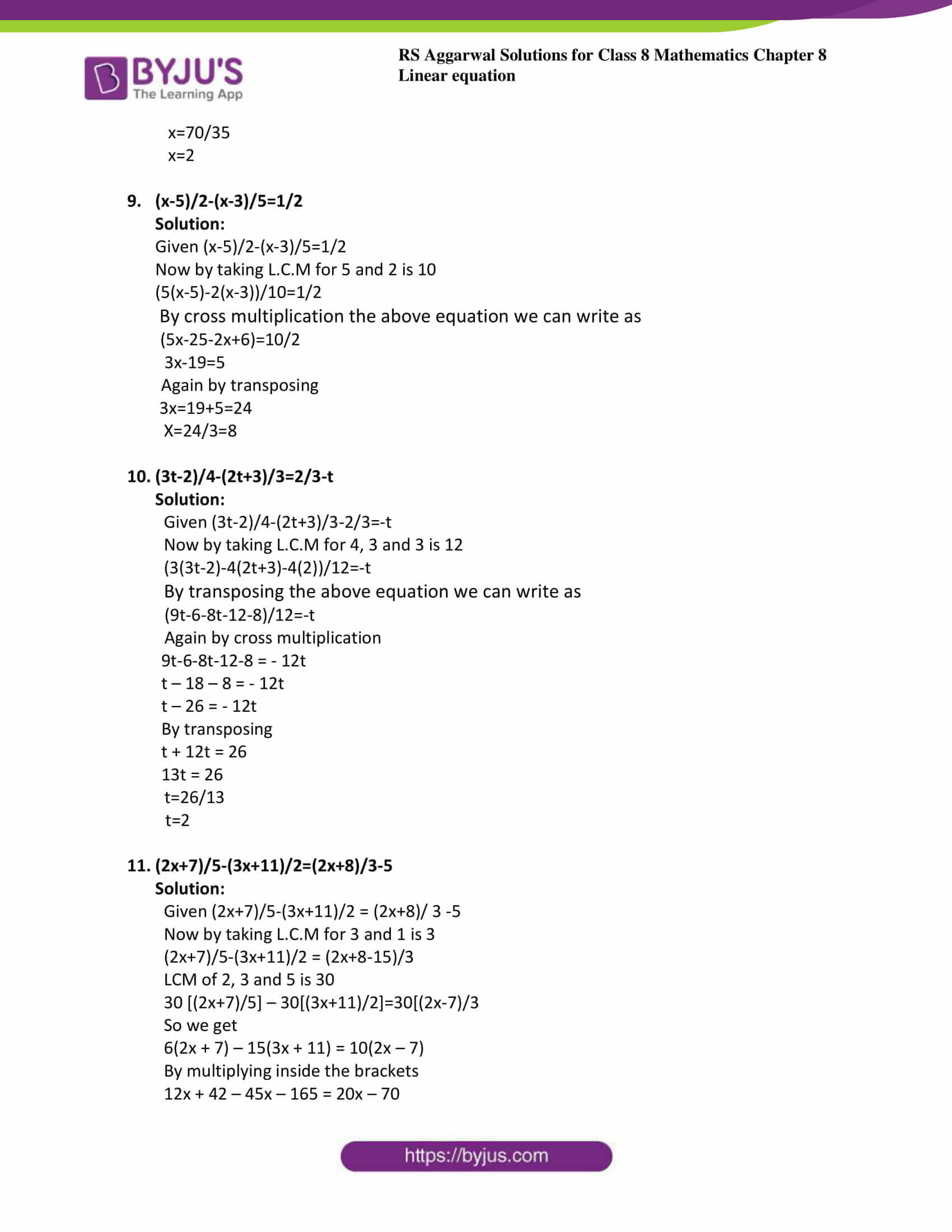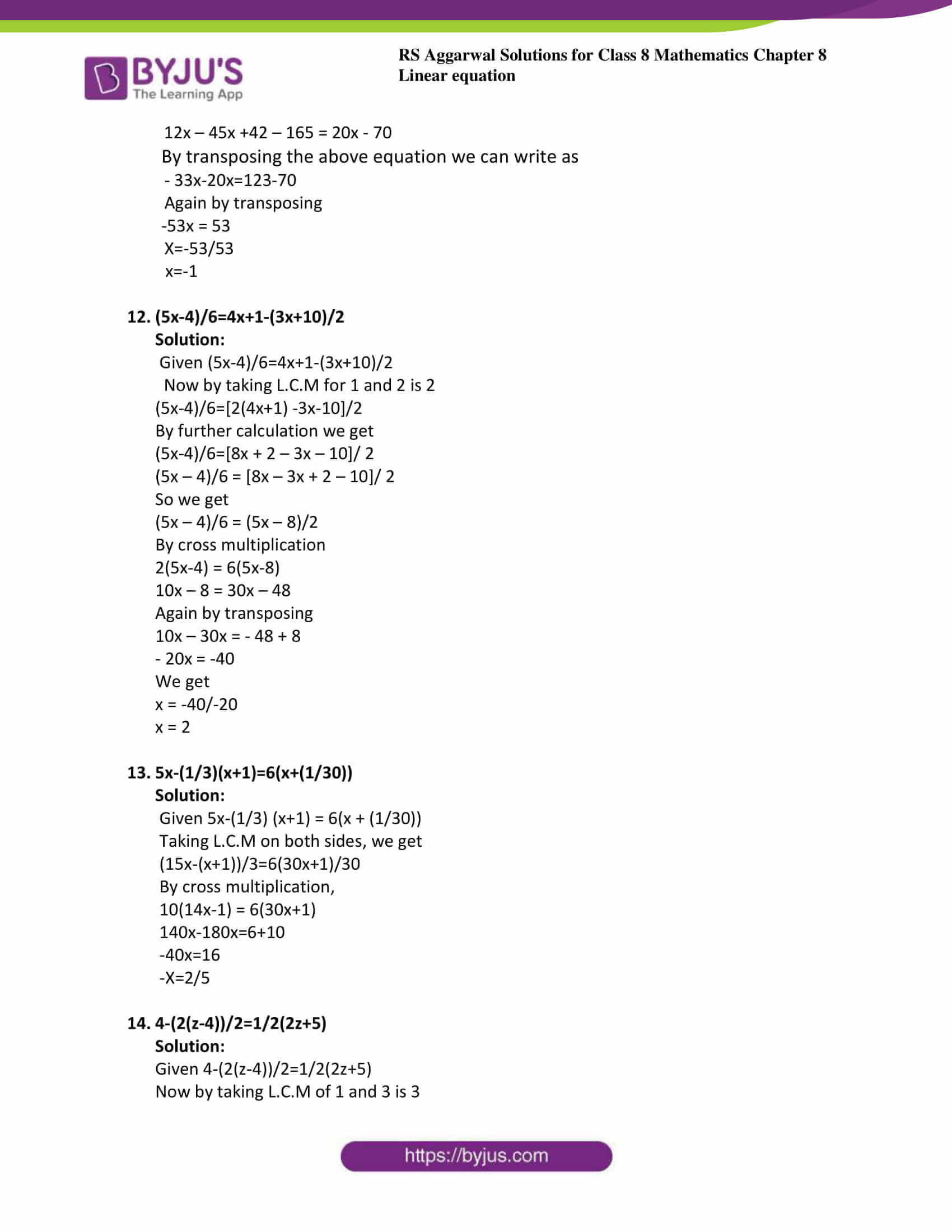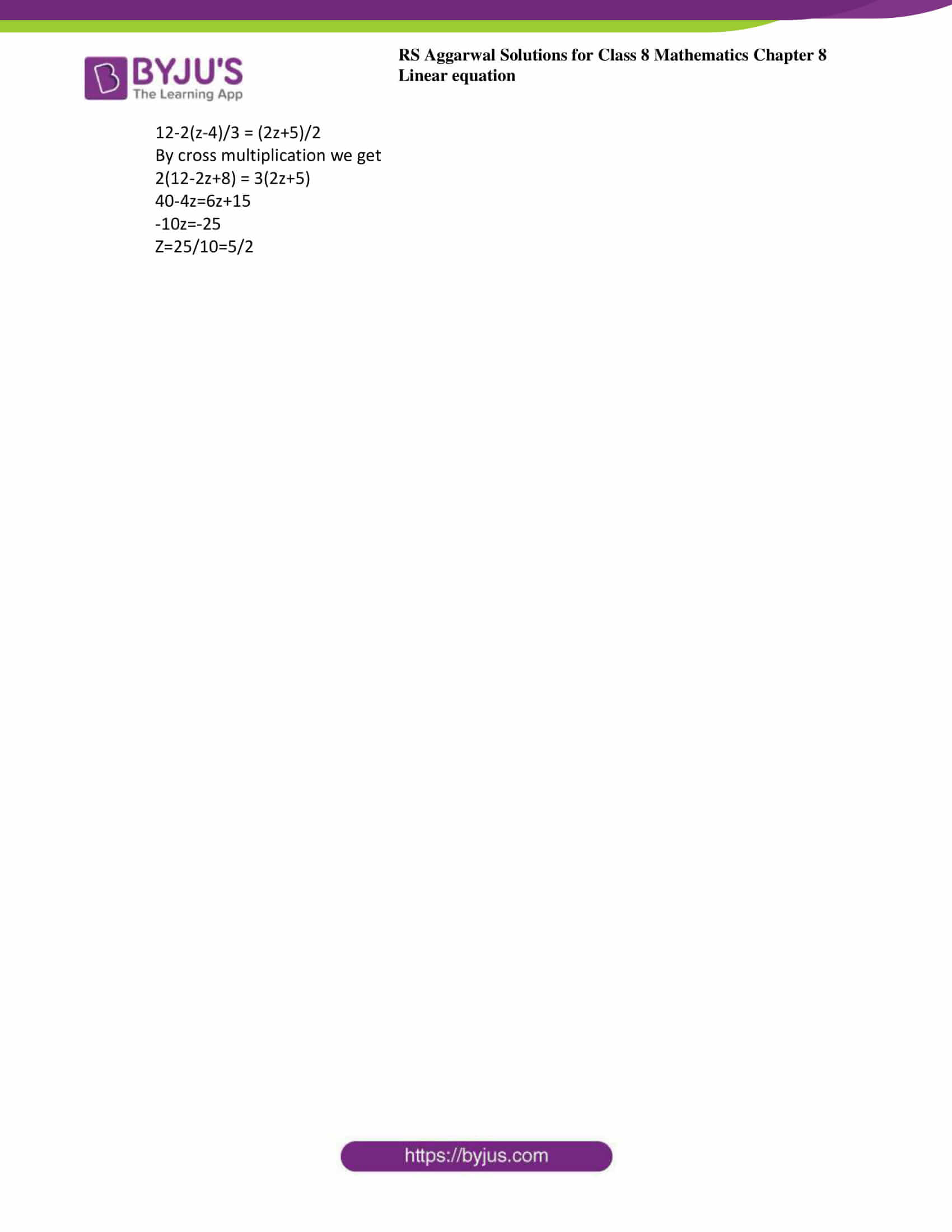### Access answers to Maths RS Aggarwal Solutions for Class 8 Chapter 8 – Linear Equations Exercise 8A

Solve:

1. 8x+3=27+2x

Solution:

Given 8x+3=27+2x

By transposing the above equation we can write as

8x-2x=27-3

6x=24

By cross multiplication

x=24/6=4

2. 5x+7=2x-8

Solution:

Given 5x+7=2x-8,

By transposing the above equation we can write as

5x-2x=-7-8

3x=-15

By cross multiplication

x=-15/3=-5

3. 2z-1=14-z

Solution:

Given 2z-1=14-z,

By transposing the above equation we can write as

2z+z=14+1

3z=15

By cross multiplication

z=15/3=5

4. 9x+5=4(x-2)+8

Solution:

Given 9x+5=4(x-2) + 8,

By transposing the above equation we can write as

9x+5=4x-8+8

9x-4x=-5

By cross multiplication

5x=-5

x=-5/5=-1

5. (7y)/5=y-4

Solution:

Given (7y)/5=y-4,

By cross multiplying the above equation we can write as

7y=5(y-4)

7y=5y-20

Again by transposing

7y-5y=-20

2y=-20

y=-20/2=-10

6. 3x+2/3=2x+1

Solution:

Given 3x+2/3=2x+1

By cross multiplying the above equation we can write as

3x+2=3(2x+1)

3x+2=6x+3

Again by transposing

3x-6x=3-2

-3x=1

x=-1/3

7. 15(y-4)-2(y-9)+5(y+6)=0

Solution:

Given 15(y-4)-2(y-9) + 5(y+6)=0

Now by rearranging we get

15y-60-2y+18+5y+30=0

18y-12=0

By transposing the above equation we can write as

18y=12

Again by cross multiplication

y=12/18

y=6/9=2/3

8. 3(5x-7)-2(9x-11)=4(8x-13)-17

Solution:

Given 3(5x-7)-2(9x-11) = 4(8x-13)-17

Now by rearranging we get

15x-21-18x+22=32x-52-17

-3x+1=32x-69

By transposing the above equation we can write as

35x=70

Again by cross multiplication

x=70/35

x=2

9. (x-5)/2-(x-3)/5=1/2

Solution:

Given (x-5)/2-(x-3)/5=1/2

Now by taking L.C.M for 5 and 2 is 10

(5(x-5)-2(x-3))/10=1/2

By cross multiplying the above equation we can write as

(5x-25-2x+6)=10/2

3x-19=5

Again by transposing

3x=19+5=24

x=24/3=8

10. (3t-2)/4-(2t+3)/3=2/3-t

Solution:

Given (3t-2)/4-(2t+3)/3-2/3=t

Now by taking L.C.M for 4, 3 and 3 is 12

(3(3t-2)-4(2t+3)-4(2))/12=-t

By transposing the above equation we can write as

(9t-6-8t-12-8)/12=-t

Again by cross multiplication

9t – 6 – 8t – 12 – 8 = – 12t

t – 18 – 8 = -12t

t – 26 = – 12t

Again by transposing

t + 12t = 26

13t = 26

t=26/13

t=2

11. (2x+7)/5-(3x+11)/2=(2x+8)/3-5

Solution:

Given (2x+7)/5-(3x+11)/2 = (2x+8)/3-5

Now by taking L.C.M for 3 and 1 is 3

(2x+7)/5-(3x+11)/2 =(2x+8-15)/3

LCM of 5, 2 and 3 is 30

30[(2x+7)/5] – 30[(3x+11)/2] = 30[(2x-7)/3]

6(2x+7) – 15(3x+11) = 10(2x-7)

By further calculation

12x+42-45x-165=20x-70

12x-45x+42-165=20x-70

-33x-123=20x-70

By transposing the above equation we can write as

-33x-20x=123-70

Again by transposing

x=-53/53

x=-1

12. (5x-4)/6=4x+1-(3x+10)/2

Solution:

Given (5x-4)/6=4x+1-(3x+10)/2

Now by taking L.C.M for 1 and 2 is 2

5x-4/6 = [2(4x+1) -3x-10]/2

By further calculation we get

5x-4/6=[8x+2-3x-10]/2

(5x-4)/6=(5x-8)/2

By cross multiplication

2(5x-4)=6(5x-8)

10x-8=30x-48

So we get

10x-30x=-48+8

-20x=-40

Again by transposing,

x=-40/-20=2

13. 5x-(1/3)(x+1)=6(x+(1/30))

Solution:

Given 5x-(1/3) (x+1) = 6(x + (1/30))

Taking L.C.M on both sides, we get

(15x-(x+1))/3=6(30x+1)/30

By cross multiplication,

10(14x-1) = 6(30x+1)

140x-180x=6+10

-40x=16

-x=2/5

14. 4-(2(z-4))/2=1/2(2z+5)

Solution:

Given 4-(2(z-4))/2=1/2(2z+5)

Now by taking L.C.M of 1 and 3 is 3

12-2(z-4)/3 = (2z+5)/2

By cross multiplication we get

2(12-2z+8) = 3(2z+5)

40-4z=6z+15

-10z=-25

z=25/10=5/2

Exercise 8B

Exercise 8C

## RS Aggarwal Solutions for Class 8 Maths Chapter 8 – Linear Equations Exercise 8A

Exercise 8A of RS Aggarwal Solutions for Chapter 8, Linear Equations deals with the topics related to equations and rules for solving a linear equation. Some of the topics focused prior to exercise 8A include the following.

• Definition of equation
• Rules for solving a linear equation
• Transposing

The RS Aggarwal Solutions can help the students in practising and learning each and every concept as it provides solutions to all questions asked in the RS Aggarwal textbook. Those who aim to score high in the Maths of class 8 are advised to practice all the questions present in RS Aggarwal as many times as possible.